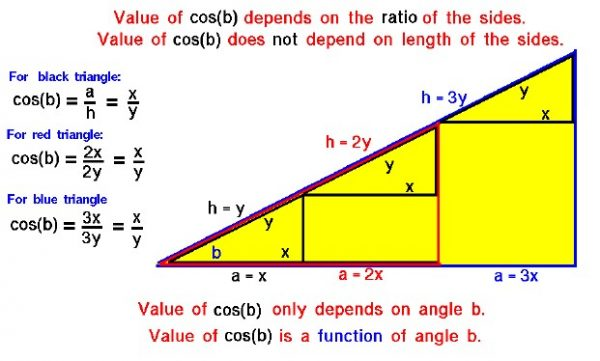# Idea of FunctionsAn important mathematical concept is the idea of function – the value of one variable in a problem depends uniquely on the value of another variable in the problem. Changing the value of one variable produces a change in the value of the other variable that is exactly the same every time you change the first variable.

## Exponential and Logarithmic Function

Some problems and relations occur so often in math and science that we assign special names to the function. The exponential function relates a variable to the number of times another variable is used as a multiplier, even if the number of times is not an integer. The logarithmic function is the inverse of the exponential; it gives the number of times that you must multiply a variable by itself to get a given number. The trigonometric functions sine, cosine, and tangent relate the various sides and angles of a triangle.

## Trigonometric Function

We can learn about functions by looking at some examples. The trigonometric functions are defined in terms of the ratio of the length of the sides of a right triangle and the claim is made that this ratio depends only on the the value of the angle formed by two sides of the triangle and not on the length of any one side. On this page we show a simple example which demonstrates this fact.

We are going to use the cosine of the angle in this example, but a similar example could be made using the sine or the tangent. Consider the large yellow triangle on the figure. It contains several other similar triangles which we will be using. All of the triangles are right triangles and all have the same angle b at the lower left corner.

The black triangle is the smallest triangle and has an hypotenuse “h” of length “y” and an adjacent side “a” equal to “x”. The length of “x” is arbitrary, but the value of “y” is not arbitrary, because the ratio of x to y, (x/y), is defined to be the cosine of “b” and this value is fixed by “b”. For the black triangle, the cosine of “b” is equal to x divided by y.

Now let us consider the red triangle. It was formed by doubling the the size of sides of the black triangle. For the red triangle, the length of the hypotenuse “h” is 2y, twice the hypotenuse of the black triangle. Similarly, the adjacent side “a” is equal to 2x relative to the black triangle. Both the red triangle and the black triangle have the same angle “b”, in blue. For the red triangle, the cosine of “b” is equal to 2x divided by 2y. Dividing out the “2’s” gives x divided by y, the same value as the black triangle.

Finally, let us consider the blue triangle. It was formed by tripling the the size of sides of the black triangle. For the blue triangle, the length of the hypotenuse “h” is 3y, and the adjacent side “a” is equal to 3x. For the blue triangle, the cosine of “b” is equal to 3x divided by 3y. Dividing out the “3’s” gives x divided by y, the same value as the black triangle and the red triangle. If we quadruple or increase the size of the sides by any multiplier, the ratio of the adjacent side to the hypotenuse stays the same x divided by y. The value of cosine “b” just depends on the value of “b” and not the length of the sides that form “b”. Scientists call this type of dependence a function. The ratio of the adjacent side to the hypotenuse is a function of the angle “b”.

## Angle of a Right Triangle

Since the cosine, and the sine and tangent, are all functions of the angle “b”, we can determine (measure) the ratios once and produce tables of the values of the sine, cosine, and tangent for various values of “b”. Later, if we know the value of an angle in a right triangle, the tables will tell us the ratio of the sides of the triangle. If we know the length of any one side, we can solve for the length of the other sides. Or if we know the ratio of any two sides of a right triangle, we can find the value of the angle between the sides. We can use the tables to solve problems. Some examples of problems involving triangles and angles include the descent of a glider, the torque on a hinge, the operation of the Wrights’ lift, and drag balances, and determining the lift to drag ratio for an aircraft.

Provide feedback